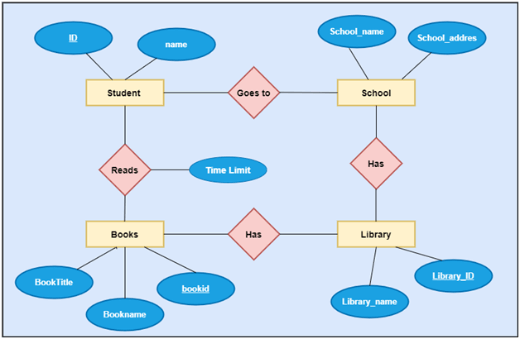# What is ER (Entity Relationship Diagram)

Today, there is a demand for Web development Skills; everybody is developing Web-based application whether it is in Java, Python, Ruby, etc. but at the end of the day we need to store the data inside the database. So, how this data will be stored, and what will be the relationship between the tables we show it using ER Diagrams, and then we can map these to a relational schema.

## What is ER Diagram?

ER Diagram is a way to design the conceptual design of the database. By conceptual it means, what will be the entities, what we will the relationship between those entities, what information of entities we need to store in the database.
So, to represent this information in the form of a diagram we use ER Diagram which acronyms to Entity Relationship Diagram which is nothing but shows the relationship between the Entities (which are objects) in the system.

## Why ER diagram is required in the application development process?

When we are building any application as a software developer we need to follow some cycle i.e. Software Development Life cycle which has some Phases in that before actual coding begins one phase is there called the Design Phase where we need to draw some diagrams so that we get a clear understanding of what we are going to implement in the application.
So, we need to draw many diagrams, from that diagrams one is ER diagram from which get the idea of what information of entities and relationship we will store in the database. The following are the reasons why ER is required:

• We would get a clear understanding of the design of the system.
• With the help of it, we can easily map it to a relational schema.
• This would help us to create a logical view of the system.

These are some good reasons why ER is required in the application development process.

## What are the Components of ER?

•  Entity: It is a real-world object in the system for e.g.: it can be Student, Computer, Faculty, etc. Entities can be of two types.
1. Physical: Student, Book
2. Logical: Account
• Entity Set: When we group similar entities it is Entities Set. E.g.: All Students will be included in Student Entity. It is represented using Rectangle.
•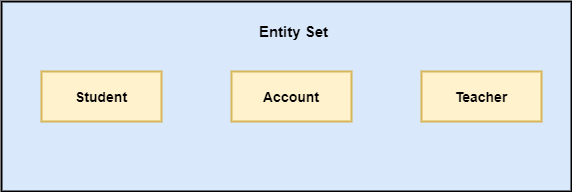Relationship: If there is an association or connection between the Entity Sets we use relationships. In common words, we can understand it like Verb. E.g.: Poet Read Novel. Here Read (Verb) is the relationship between Poet and Novel.
• Relationship Set: It is a set of Similar Relationships in the system. It is represented using Diamond.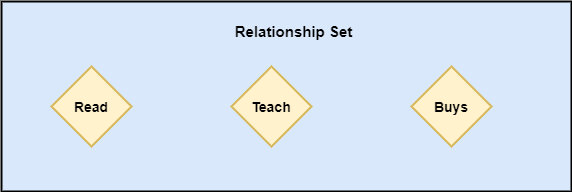• Attributes: It is a state of an entity. For e.g.: The Student has attributes like name, roll no, class, division, and etc. It is represented using the Oval/Ellipse shape.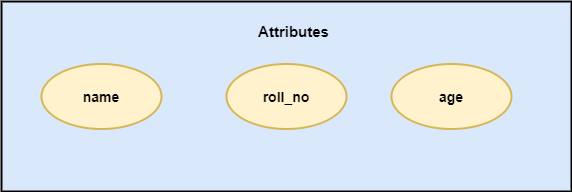## The classification of Attributes

• Composite Attributes: Those attributes that can be divided further. Like a Person can have more than one Mobile Numbers.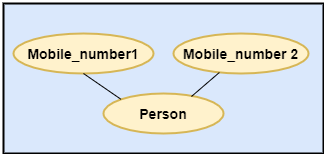• Simple Attributes: Those attribute which cannot be divided further. Like a Salary.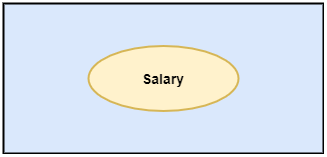• Single Valued Attribute: It takes almost one value for each entity in the system. Like Date of Birth.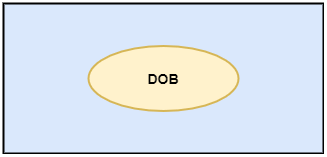• Multi-valued Attribute: It takes more than one value. Like we can have more than one email ID. It is represented using a double ellipse.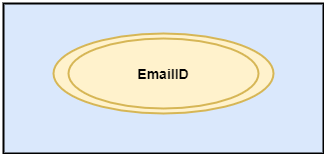• Stored Attribute: It is the attribute whose value cannot be changed. Like Date of Death – It cannot be changed.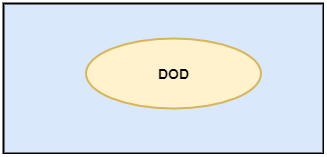• Derived Attributes: using one attribute if we can derive another attribute is Derived Attributes. Like we can calculate the Percentage-based on marks. It is represented using a dotted ellipse.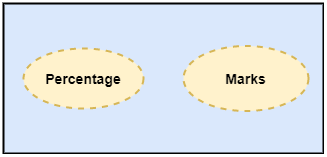• Key Attribute: It is an attribute that uniquely identifies the entity in the system. Like Student_ID. It is shown by using underline.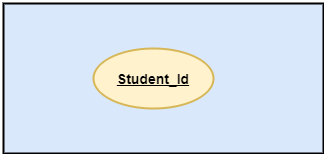• Descriptive Attribute: If we need any attribute to describe the relationship set we use Descriptive Attribute.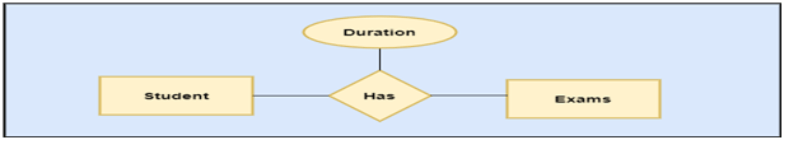## How to create a simple ER Diagram with an example?

Here, we will see How to create a simple ER Diagram with the help of the components that we discussed above. Let’s create a simple ER diagram For Students System.

It will have 4 entities

• Students
• School
• Library
• Books

It will have 4 relationships.

• Student Goes to School